#Function Repository Resource:

# Cofactor

Get a cofactor of a matrix

Contributed by: Wolfram Staff
 ResourceFunction["Cofactor"][m,{i,j}] gives the (i,j) cofactor of the matrix m.

## Examples

### Basic Examples

Here is the 1, 2 cofactor of a 3×3 matrix:

 In:=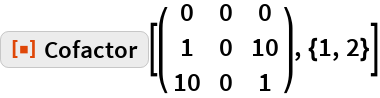Out=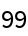The positively signed cofactor of the top left-hand corner of an array:

 In:=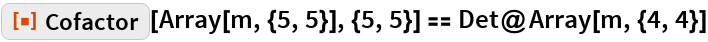Out=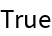### Scope

Define a random 4×4 matrix:

 In:=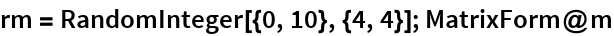Out=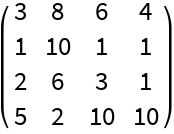Here is its determinant:

 In:=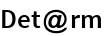Out=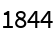Expand the determinant along the first row:

 In:=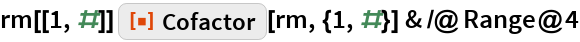Out=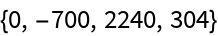Adding up gives the determinant again:

 In:=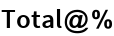Out=